Homework Help Question & Answers

# 3) Pol the diferential equation: (a) The point o -1 is an ordinary point. Compute the recursion formula for the coefficients of the power series solution centered at zo- -1 and use it to compute...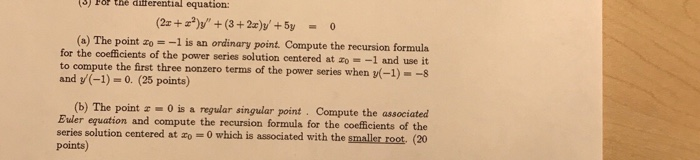3) Pol the diferential equation: (a) The point o -1 is an ordinary point. Compute the recursion formula for the coefficients of the power series solution centered at zo- -1 and use it to compute the first three nonzero terms of the power series when (-1)--s and y(-1)-0. (25 points) (b) The point 0 is a regular singular point Compute the associated Buler equation and compute the recursion formula for the coefficients of the series solution centered at o 0 which is associated with the smaller root. (20 points)

#### Homework Answers

Answer #1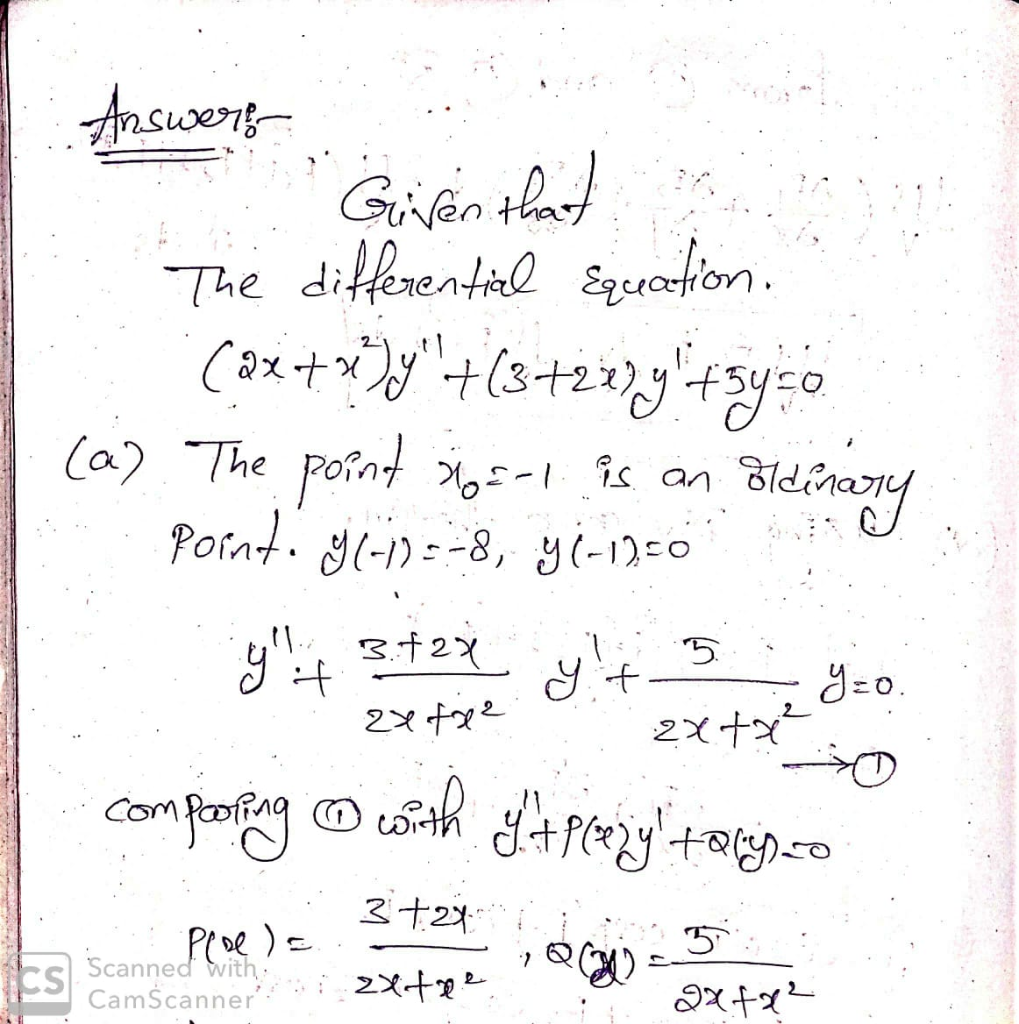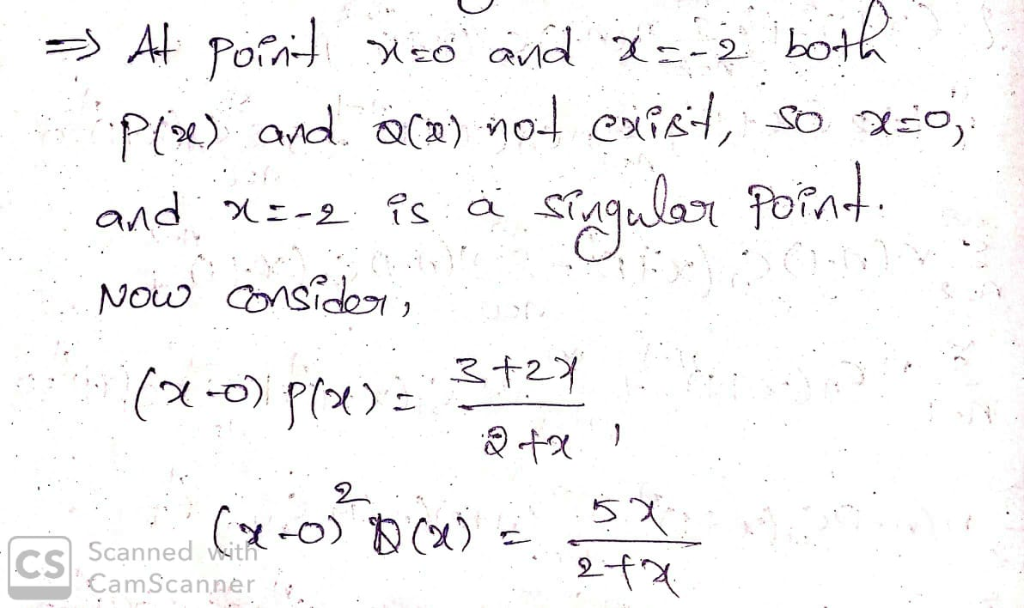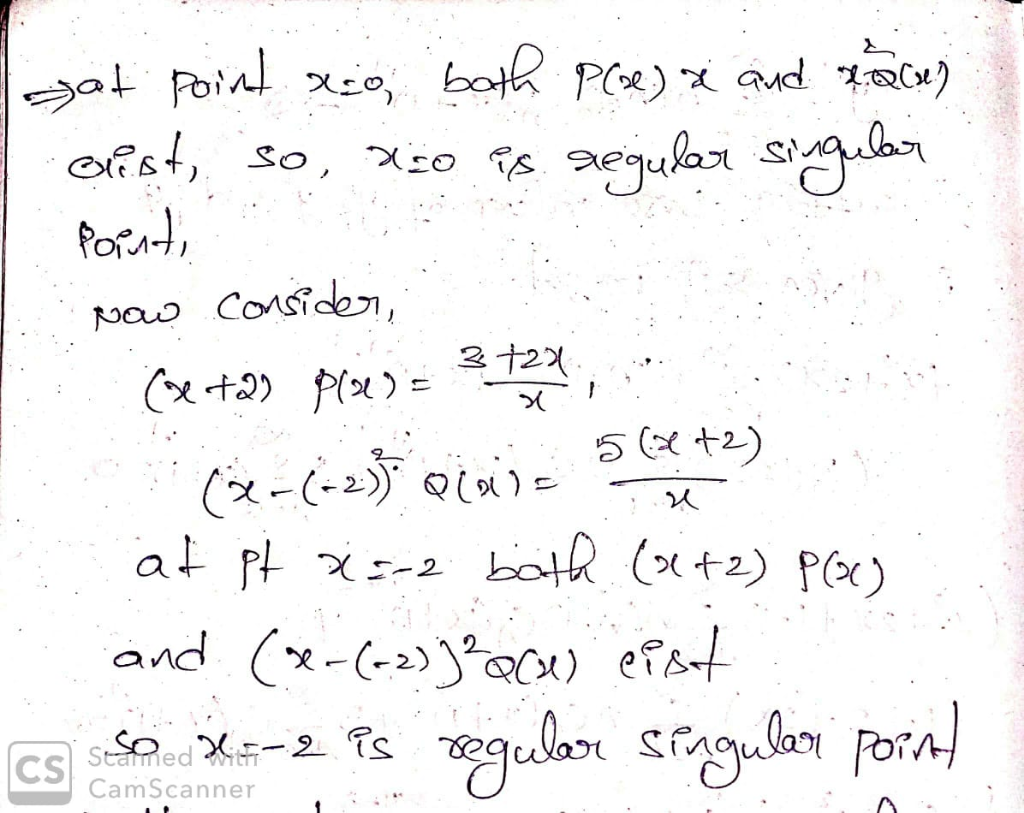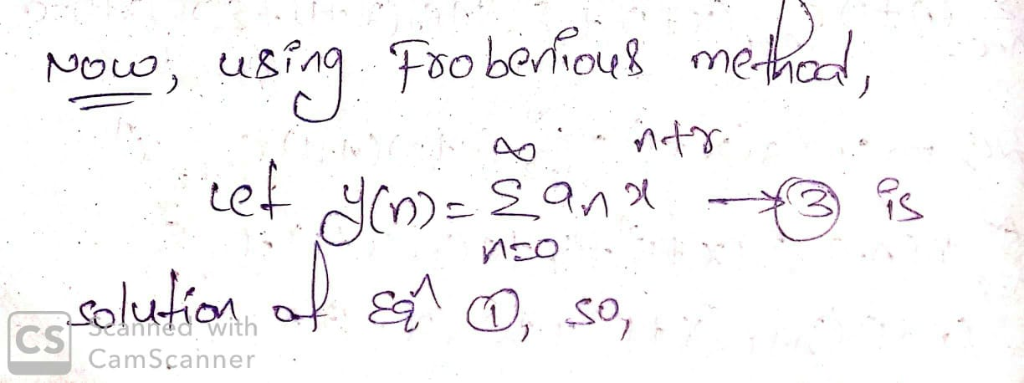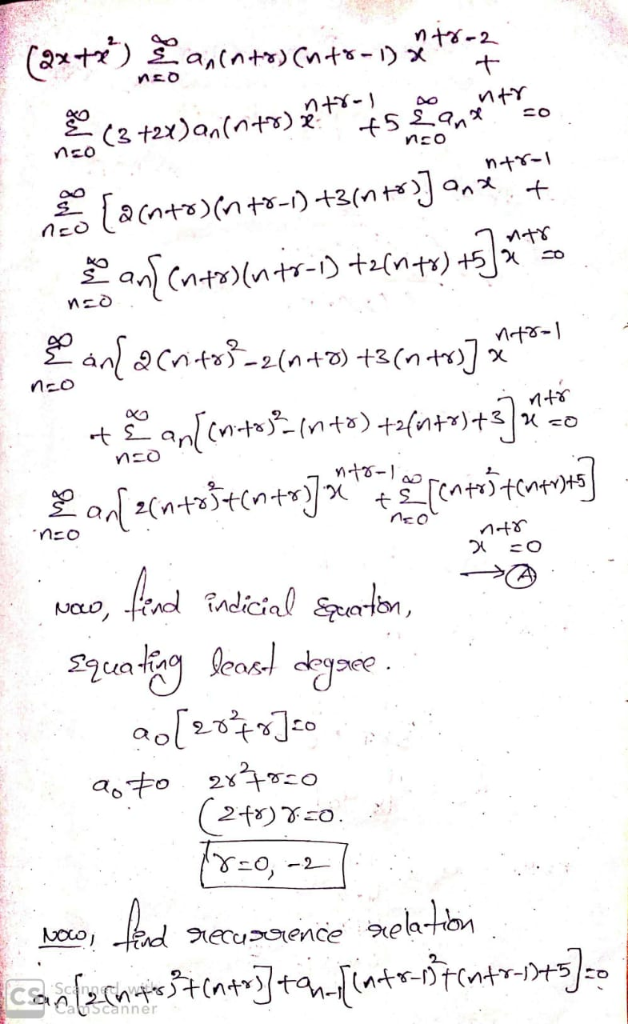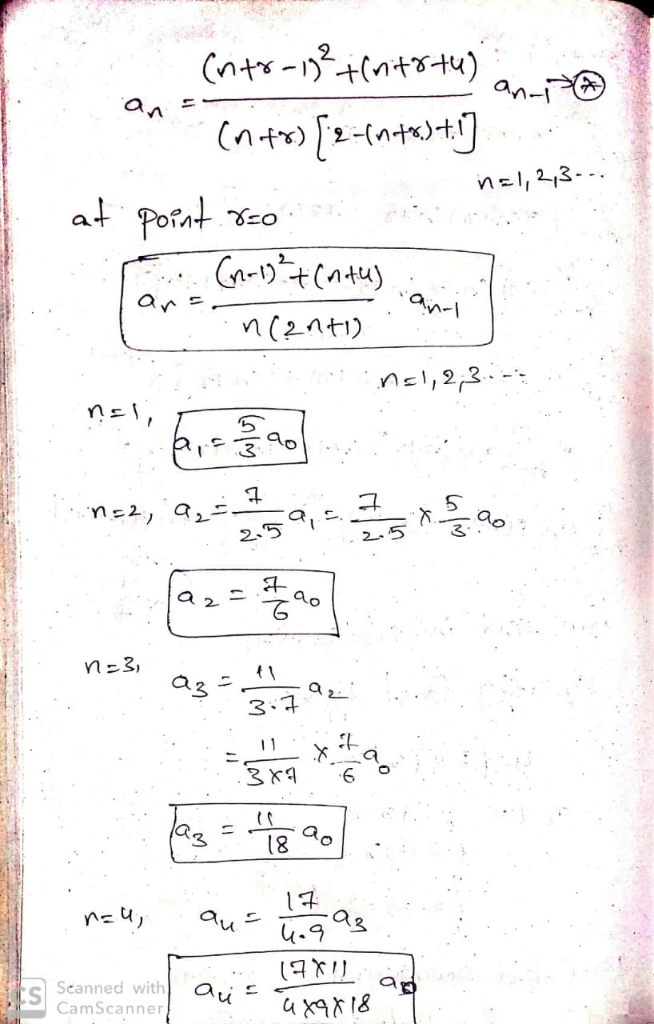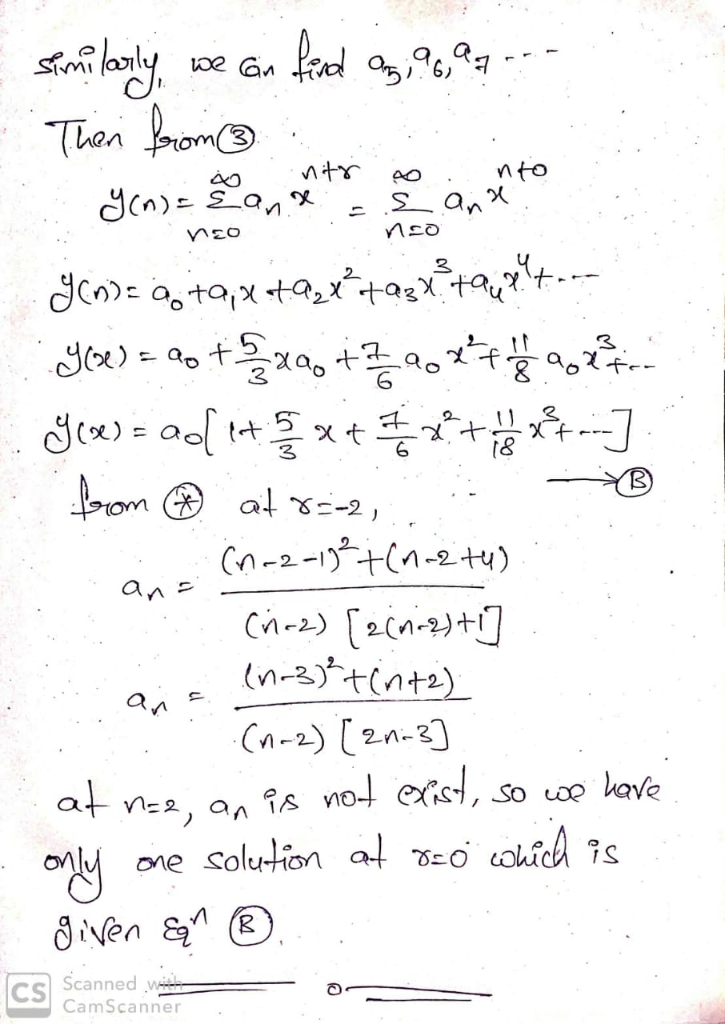Know the answer?
Your Answer:

#### Post as a guest

Your Name:

What's your source?

#### Earn Coin

Coins can be redeemed for fabulous gifts.

Not the answer you're looking for? Ask your own homework help question. Our experts will answer your question WITHIN MINUTES for Free.
Similar Homework Help Questions
• ### B only It’s review question, I need this as soon as possible. Thank you 3) Pol the diferential equation: (a) The point o -1 is an ordinary point. Compute the recursion formula for the coeffici...B only It’s review question, I need this as soon as possible. Thank you 3) Pol the diferential equation: (a) The point o -1 is an ordinary point. Compute the recursion formula for the coefficients of the power series solution centered at zo- -1 and use it to compute the first three nonzero terms of the power series when (-1)--s and y(-1)-0. (25 points) (b) The point 0 is a regular singular point Compute the associated Buler equation and compute...

• ### It’s review question, I need this as soon as possible. Thank you 3) For thè diferential equation: (a) The point zo =-1 is an ordinary point. Compute the recursion formula for the coefficients of...It’s review question, I need this as soon as possible. Thank you3) For thè diferential equation: (a) The point zo =-1 is an ordinary point. Compute the recursion formula for the coefficients of the power series solution centered at zo- -1 and use it to compute the first three nonzero terms of the power series when -1)-s and v(-1)-0. (25 points) (b) The point z = 0 is a regular singular point . Compute the associated Euler equation and compute...

• ### (6) (18 points) For the differential equation: Compute the recursion formula for the coefficients...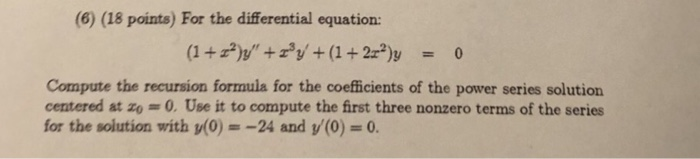(6) (18 points) For the differential equation: Compute the recursion formula for the coefficients of the power series solution centered at zo 0.Use it to compute the first three nonzero terms of the series for the solution with y(0) =-24 and y(0) = 0. (6) (18 points) For the differential equation: Compute the recursion formula for the coefficients of the power series solution centered at zo 0.Use it to compute the first three nonzero terms of the series for the...

• ### (4) (12 points)For the differential equation: Compute the recursion formula for the coefficients of the power series solution centered at o 0 and use it to compute the first three nonzero terms of...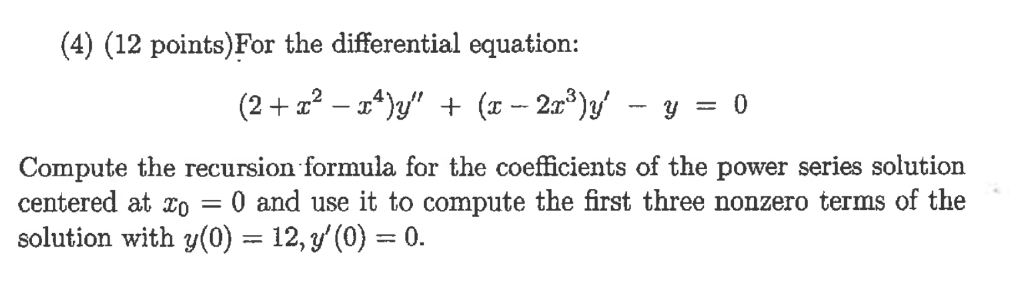(4) (12 points)For the differential equation: Compute the recursion formula for the coefficients of the power series solution centered at o 0 and use it to compute the first three nonzero terms of the solution with y(0) 12, y'(0)0. (5) (12 points)For the equation y" - 5ty -7y 0 (t>0), (t)t is a solution (a) Use the method of Reduction of Order to obtain a second, independent solution. (b) Solve the equation directly, using that it is an Euler Equation....

• ### 1) Show that zo-0 is a regular singular point for the diferenta equation Zo = 0 is a regular sing...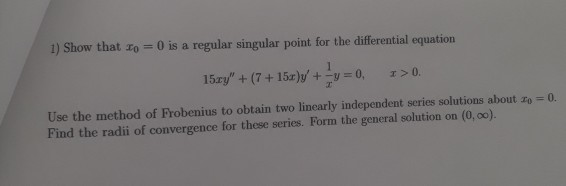please show the recurrence formula 1) Show that zo-0 is a regular singular point for the diferenta equation Zo = 0 is a regular singular point for the differential equation 15ェy" + (7 + 15r)y, +-y = 0, x>0. Use the method of Frobenius to obtain two linearly independent series solutions about zo Find the radii of convergence for these series. Form the general solution on (0, 0o). 0. 1) Show that zo-0 is a regular singular point for the...

• ### 2. Find power series solutions y z" Σ anr" of the following equation centered at 0...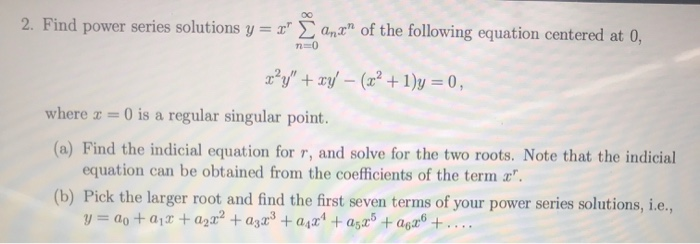2. Find power series solutions y z" Σ anr" of the following equation centered at 0 where-0 is a regular singular point. (a) Find the indicial equation for r, and solve for the two roots. Note that the indicial equation can be obtained from the coefficients of the term Pick the larger root and find the first seven terms of your power series solutions, i.e., (b)

• ### Use a power series centered about the ordinary point x0 = 0 to solve the differential equation (x − 4)y′′ − y′ + 12xy =...

Use a power series centered about the ordinary point x0 = 0 to solve the differential equation (x − 4)y′′ − y′ + 12xy = 0 Find the recurrence relation and at least the first four nonzero terms of each of the two linearly inde- pendent solutions (unless the series terminates sooner). What is the guaranteed radius of convergence?

• ### Question 3 Consider the ordinary differential equation (ODE) 2xy" + (1 + x)y' + 3y =...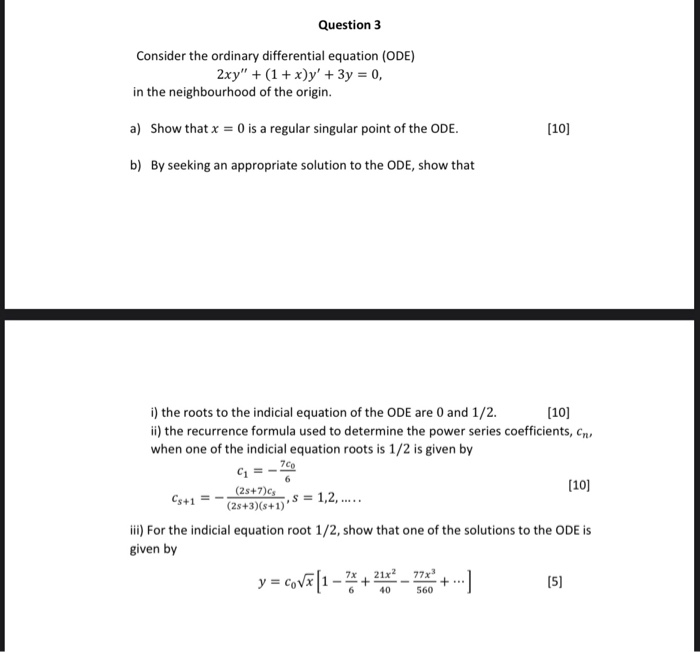Question 3 Consider the ordinary differential equation (ODE) 2xy" + (1 + x)y' + 3y = 0, in the neighbourhood of the origin. a) Show that x = 0 is a regular singular point of the ODE. (10) b) By seeking an appropriate solution to the ODE, show that G=- (10) i) the roots to the indicial equation of the ODE are 0 and 1/2.  ii) the recurrence formula used to determine the power series coefficients, ens when one...

• ### Need some help with SERIES SOLUTION - 2nd ORDER EQUATION For the differential equation,          (1) a. Calculate the indicial equation for the power series solution (Answer in a quadratic polynomial...

Need some help with SERIES SOLUTION - 2nd ORDER EQUATION For the differential equation,          (1) a. Calculate the indicial equation for the power series solution (Answer in a quadratic polynomial in terms of c.) b. Calculate the solutions of the indicial equation found above. c. Calculate the point from the above equation (1) as i. ORDINARY POINT ii. REGULAR SINGULAR POINT iii. IRREGULAR SINGULAR POINT We were unable to transcribe this imagey-Σ@m(z _ 4)nte We were unable to transcribe this...

• ### (Example 6.3.2) Find the general solution of the differential equation 5. in series centered at the regular singular po...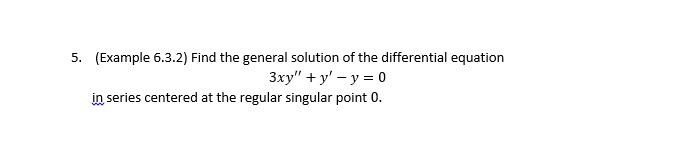(Example 6.3.2) Find the general solution of the differential equation 5. in series centered at the regular singular point 0

Free Homework App

Scan Your Homework
to Get Instant Free Answers
Need Online Homework Help?

Get Answers For Free
Most questions answered within 3 hours.